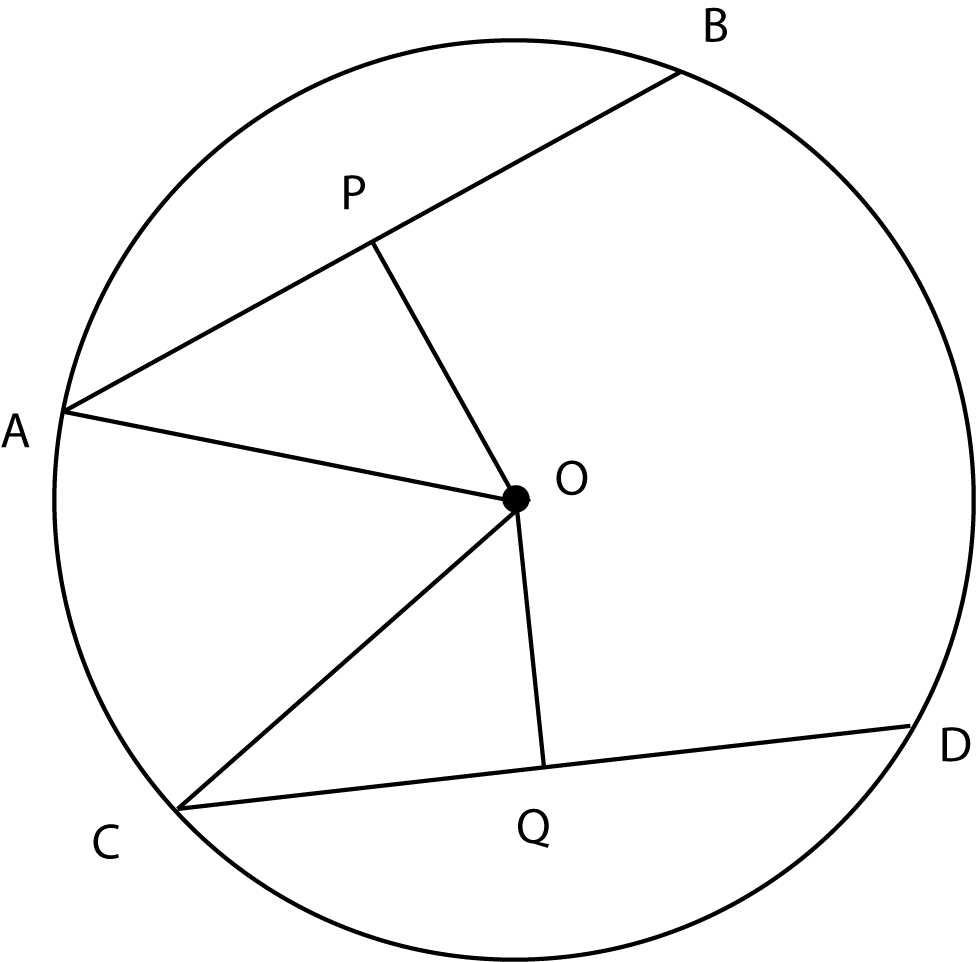### Prove: AP = CQDefinition
Equal arcs have equal chords
AB = AP + PB and CD = CQ + QD
AP + AP = CQ + CQ
If a diameter is perpendicular to a chord, it bisects the chord
OP ⟂ AB and OQ ⟂ CD
Substitution
AP = CQ
OP bisects AB and OQ bisects CD
Substitution
Algebra
AP + PB = CQ + QD
AP = PB and CQ = QD
AB = CD
Given